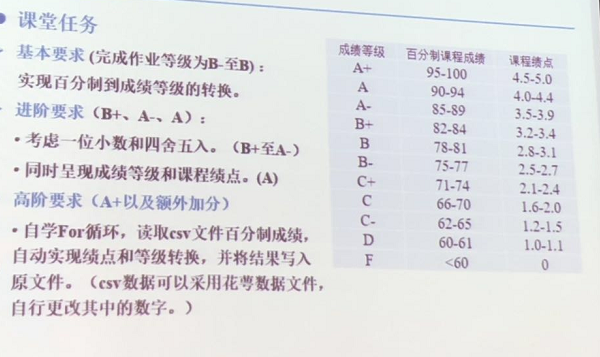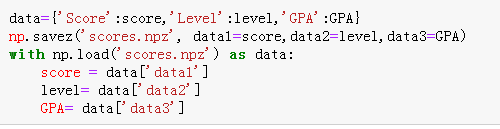python如何读取一个csv进行操作再将结果写入原文件？``````import numpy as np
level = []#创建记录等级的空列表
GPA = []#创建记录绩点的空列表
for i in score:
gpa = round(i/10-5,1)
if 95<=i<=100:
print("成绩:{},等级:{},绩点:{}".format(i,'A+',gpa)
level.append('A+')
GPA.append(gpa)
elif 90<=i<95:
print("成绩:{},等级:{},绩点:{}".format(i,'A',gpa)
level.append('A')
GPA.append(gpa)
elif 85<=i<90:
print("成绩:{},等级:{},绩点:{}".format(i,'A-',gpa)
level.append('A-')
GPA.append(gpa)
elif 82<=i<85:
print("成绩:{},等级:{},绩点:{}".format(i,'B+',gpa)
level.append('B+')
GPA.append(gpa)
elif 78<=i<82:
print("成绩:{},等级:{},绩点:{}".format(i,'B',gpa)
level.append('B')
GPA.append(gpa)
elif 75<=i<78:
print("成绩:{},等级:{},绩点:{}".format(i,'B-',gpa)
level.append('B-')
GPA.append(gpa)
elif 71<=i<75:
print("成绩:{},等级:{},绩点:{}".format(i,'C+',gpa)
level.append('C+')
GPA.append(gpa)
elif 66<=i<71:
print("成绩:{},等级:{},绩点:{}".format(i,'C',gpa)
level.append('C')
GPA.append(gpa)
elif 62<=i<66:
print("成绩:{},等级:{},绩点:{}".format(i,'C-',gpa)
level.append('C-')
GPA.append(gpa)
elif 60<=i<62:
print("成绩:{},等级:{},绩点:{}".format(i,'D',gpa)
level.append('D')
GPA.append(gpa)
elif i<60:
print("成绩:{},等级:{},绩点:{}".format(i,'F',0)
level.append('F')
GPA.append(0)

``````Python读取csv文件后str转换为float

-------------------------------------------code--------------------------------- #coding:utf-8 import csv import xlwt #新建excel文件 myexcel = xlwt.Workbook() #新建sheet页 mysheet = myexcel.add_sheet("testsheet") csvfile = open("data.csv","r") #读取文件信息 reader = csv.reader(csvfile) l = 0 #通过循环获取单行信息 for line in reader: r = 0 #通过双重循环获取单个单元信息 for i in line: #通过双重循环写入excel表格 x=0 #将第六行第二列的str转换为float if l > 6: if r >= 1: #x=float(i) x = float(i) #print(i) if x > 160: print(l,r) mysheet.write(l,r,"high") else: mysheet.write(l,r,i) r=r+1 l=l+1 #最后保存到excel myexcel.save("myexcel.xls") -------------------------------------------code--------------------------------- 如上，x=float(i)处报错ValueError:could not convert string to float: '-' 此处数据类似 16.02 如何解决。

Python 读取MYSQL数据并插入到CSV文件相应位置

python csv写入，为啥头信息会重复

Python中进行了csv格式数据的筛选，如何将这些筛选数据进行另存为新的csv

python3实现根据csv文件动态建表

pandas关于csv的读取和写入问题

python3循环写入文件的问题

![图片说明](https://img-ask.csdn.net/upload/201608/09/1470722627_895105.jpg) 如图所示，在excel文件中，我想把第一个txt的数据写入excel的第一列，把第二个txt的数据写入excel的第二列中？现在我只能把两个txt文件的数据全部放在一列中，如何修改才能达到目的呢？码代如下： ``` #include <iostream> #include <fstream> #include <vector> #include <windows.h> #include <string> using namespace std; char* WcharToChar(const wchar_t* wp) { char *m_char; int len= WideCharToMultiByte(CP_ACP,0,wp,wcslen(wp),NULL,0,NULL,NULL); m_char=new char[len+1]; WideCharToMultiByte(CP_ACP,0,wp,wcslen(wp),m_char,len,NULL,NULL); m_char[len]='\0'; return m_char; } wchar_t* CharToWchar(const char* c) { wchar_t *m_wchar; int len = MultiByteToWideChar(CP_ACP,0,c,strlen(c),NULL,0); m_wchar=new wchar_t[len+1]; MultiByteToWideChar(CP_ACP,0,c,strlen(c),m_wchar,len); m_wchar[len]='\0'; return m_wchar; } wchar_t* StringToWchar(const string& s) { const char* p=s.c_str(); return CharToWchar(p); } int main() { const string fileform = "*.txt"; const string perfileReadPath = "Data"; const int perclass_sample = 20; string fileReadPath; int nLine = 0; string buf; vector<float> descriptors; int j = 0; ofstream file("data_file.csv"); fileReadPath = perfileReadPath + "/" + fileform; HANDLE hFile; LPCTSTR lpFileName = StringToWchar(fileReadPath); WIN32_FIND_DATA pNextInfo; hFile = FindFirstFile(lpFileName, &pNextInfo); if(hFile == INVALID_HANDLE_VALUE) { exit(-1); } do { if(pNextInfo.cFileName == '.') continue; j++; printf("%s\n",WcharToChar(pNextInfo.cFileName)); ifstream src_data(perfileReadPath + "/" + WcharToChar(pNextInfo.cFileName)); while( src_data ) { if( getline( src_data, buf ) ) { nLine++; descriptors.push_back( atof(buf.c_str()) ); } } src_data.close(); for(int i=0; i<descriptors.size();i++) { file<<descriptors[i]<<endl; } //格式化容器，清除容器内所有数据，为保存下一个txt文件数据文件做准备 descriptors.clear(); } while (FindNextFile(hFile,&pNextInfo) && j<perclass_sample); return 0; } ```

python 处理csv或excel文件时遇到空白单元格怎么处理。

【python】用python读取txt并按规则写入excle

with open('C:/Users/czm/Desktop/历史数据记录2016-12-01 00-10-22--2017-01-31 23-10-22.csv') as csvfile: reader = csv.DictReader(csvfile) print(type(reader)) data = [] # 取出文件的表头信息 head = reader.fieldnames for row in reader: # 按表头信息取出（列的信息） temp = row[head] # 将取出的信息转化成字符串存储到元组中 data.append(''.join(temp)) timelist = TimerHelper.FormatTime(data) spacelist = TimerHelper.GetTimeSpace(timelist) breakpoint = Tools.GetBreakPoint(spacelist) for item in breakpoint: print(data[item], '在文件{0}{1}'.format(item + 2, '行'), '缺失{0}{1}'.format(item, '条数据')) for item in reader: print(item) 第一次for循环遍历完成后再次循环无法输出，是指针已经指到最后一行了么？可以重新遍历么？

python 爬取后存入csv，出现一堆未识别问号

String s = new String(" a ") 到底产生几个对象？

Linux面试题（2020最新版）

JVM内存结构和Java内存模型别再傻傻分不清了

loonggg读完需要3分钟速读仅需 1 分钟大家好，我是你们的校长。我之前讲过，这年头，只要肯动脑，肯行动，程序员凭借自己的技术，赚钱的方式还是有很多种的。仅仅靠在公司出卖自己的劳动时...

85后蒋凡：28岁实现财务自由、34岁成为阿里万亿电商帝国双掌门，他的人生底层逻辑是什么？...

MySQL数据库面试题（2020最新版）

HashMap底层实现原理，红黑树，B+树，B树的结构原理 Spring的AOP和IOC是什么？它们常见的使用场景有哪些？Spring事务，事务的属性，传播行为，数据库隔离级别 Spring和SpringMVC，MyBatis以及SpringBoot的注解分别有哪些？SpringMVC的工作原理，SpringBoot框架的优点，MyBatis框架的优点 SpringCould组件有哪些，他们...